Yoshihara, H. (2012). "Influence of the specimen depth to length ratio and lamination construction on Young's modulus and in-plane shear modulus of plywood measured by flexural vibration," BioRes. 7(1), 1337-1351.

#### Abstract

In this study, the Young’s modulus and the in-plane shear modulus of 3-, 5-, and, 7-ply Lauan wood (Shorea sp.) were determined by conducting a flexural vibration test with various specimen depth to length ratios and performing a subsequent finite element analysis (FEA). The length and depth directions of the specimen used for the vibration test coincided with the length/width and width/length directions of the plywood panel. The results obtained from the experiment and FEA revealed that the influence of specimen configuration and lamination construction did not significantly affect the measurement of the Young’s modulus. However, the results suggested that the in-plane shear modulus decreased as the depth to length ratio of the specimen decreased. The FEA result suggested that this decreasing tendency is more pronounced as the ply number decreases and the thickness of the plywood increases. A statistical analysis on the experimental results suggested that the length of the specimen must be less than 10 times the depth to reduce the influence of specimen configuration on the measured value of the in-plane shear modulus.

INFLUENCE OF THE SPECIMEN DEPTH TO LENGTH RATIO AND LAMINATION CONSTRUCTION ON YOUNG’S MODULUS AND IN-PLANE SHEAR MODULUS OF PLYWOOD MEASURED BY FLEXURAL VIBRATION

Hiroshi Yoshihara a

In this study, the Young’s modulus and the in-plane shear modulus of 3-, 5-, and, 7-ply Lauan wood (Shorea sp.) were determined by conducting a flexural vibration test with various specimen depth to length ratios and performing a subsequent finite element analysis (FEA). The length and depth directions of the specimen used for the vibration test coincided with the length/width and width/length directions of the plywood panel. The results obtained from the experiment and FEA revealed that the influence of specimen configuration and lamination construction did not significantly affect the measurement of the Young’s modulus. However, the results suggested that the in-plane shear modulus decreased as the depth to length ratio of the specimen decreased. The FEA result suggested that this decreasing tendency is more pronounced as the ply number decreases and the thickness of the plywood increases. A statistical analysis on the experimental results suggested that the length of the specimen must be less than 10 times the depth to reduce the influence of specimen configuration on the measured value of the in-plane shear modulus.

Keywords: Finite element analysis; Flexural vibration method; In-plane shear modulus; Plywood; Young’s modulus

Contact information: Contact information: a: Faculty of Science and Engineering, Shimane University, Nishikawazu-cho 1060, Matsue, Shimane 690-8504, Japan;

* Corresponding author: yosihara@riko.shimane-u.ac.jp

INTRODUCTION

Hardwood plywood is one of the most common wood products used in construction. To ensure that the design of construction is reliable and cost effective, it is essential to reliably determine the elastic properties of plywood such as its Young’s modulus and shear modulus.

The flexural vibration method is well established for the measurement of the Young’s modulus and shear modulus of solid wood and plywood (Hearmon 1958, 1966; Nakao 1984; Sobue 1986; Nakao and Okano 1987; Haines et al. 1996; Kubojima et al. 1996, 1997; Chui and Smith 1999; Divós et al. 1998, 2005; Brancheriau and Baillères 2002, 2003; Brancheriau 2006; Murata and Kanazawa 2007; Tonosaki et al. 2010; Sohi et al. 2011; Yoshihara 2012). Solid wood usually has a Young’s modulus/shear modulus ratio in a range of approximately 5 to 20 (Hearmon 1948). This ratio is larger than that of isotropic materials, which have a typical range of 2 to 3. To measure the shear modulus and Young’s modulus accurately by flexural vibration, a large value of this ratio is desirable because the shear deflection increases as the ratio increases. Consequently, the flexural vibration method is effective for measuring the shear modulus of solid wood. For plywood, the Young’s modulus/in-plane shear modulus ratio also ranges from 5 to 20 (Hearmon 1948), and, therefore, the in-plane shear modulus of plywood can be effectively measured by the flexural vibration method. In a previous study, the validity of the flexural vibration method was examined for Lauan five-ply wood by comparing the in-plane shear moduli obtained by the static bending, dynamic square-plate twisting, and static square-plate twisting methods (Yoshihara 2009a). It was found that the flexural vibration method is superior to the other three methods for measuring the in-plane shear modulus. In the previous work, however, the configurations and lamination constructions of the plywood specimens did not vary.

In plywood, the influence of the specimen configuration on the measurement of elastic constant is often enhanced by the lamination construction. For example, the influence of specimen configuration is considered when measuring the Poisson’s ratio and in-plane shear modulus of plywood by static tension tests because of the inhomogeneous distribution of the strain induced by the lamination (Yoshihara 2009b, 2010). For similar reasons, there is a concern that the Young’s modulus and in-plane shear modulus of plywood cannot be measured accurately by the flexural vibration method without considering the influences of specimen configuration and lamination construction. Nevertheless, there are few reports in the literature that examine these influences.

In this work, flexural vibration tests were performed on Lauan 3-, 5-, and 7-ply wood specimens with various depth/length ratios. The length and depth directions of the specimen used for the vibration test coincided with the length/width and width/length directions of the plywood panel, so the Young’s modulus value in the length and width directions of the plywood and the in-plane shear modulus value in the length/width plane were obtained. The validity of the method was examined by comparing its results with finite element (FE) calculations. The objective of this work was to reveal the appropriate specimen configuration for determining the Young’s modulus and in-plane shear modulus of plywood.

ELASTIC PROPERTIES DERIVED BY CLASSICAL LAMINATION THEORY

Figure 1 shows a diagram of a plywood panel. Usually, plywood is made from wood veneers of a single species. The fiber directions in alternate veneers, which are produced either by slicing or by peeling, are at right angles. Therefore, the longitudinal and tangential directions of the surface veneer coincide with the length and width directions of the plywood.

As shown in Fig. 1a, the length, width, and thickness directions of the plywood panel are defined as the xy, and z directions, respectively. The thicknesses of the surface veneer and the veneer adjacent to the surface veneer are defined as ta and tb, respectively, as shown in Fig. 1b.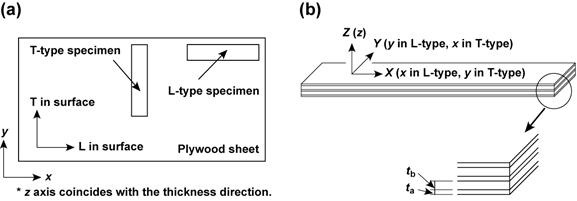Fig. 1. (a) Diagram of the plywood panel and types of specimens. (b) Definitions of XY, and Z axes

The elastic properties of plywood are often determined on the basis of classical lamination theory. The Young’s moduli in the length, width, and thickness directions of the plywood panel (which are defined as ExEy, and Ez, respectively, as shown in Fig. 1a) are derived as follows (Hearmon 1948; Takami 1964; Adams et al. 2003; Yoshihara 2009b):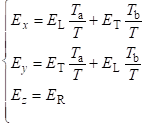(1)

where EL, ET, and ER are the Young’s moduli in the longitudinal (L), tangential (T), and radial (R) directions of the veneer, respectively. Ta and Tb are the total thicknesses of the veneers with fiber directions coincident with the surface veneer and the veneer adjacent to the surface veneer, respectively, and T is the total thickness of the plywood panel, so T = Ta + Tb.

The shear moduli in the xyyz, and xz planes of the plywood, defined as Gxy , Gyz, and Gxz, respectively, are defined by the following expressions (Adams et al. 2003):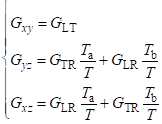(2)

where GLT, GTR, and GLR are the shear moduli in the LT, TR, and LR planes of the veneer, respectively.

The Poisson’s ratios in the xyyz, and xz planes of the plywood, defined as xyyz, and xz, respectively, are derived as follows (Hearmon 1948; Takami 1964; Adams et al. 2003; Yoshihara 2009b):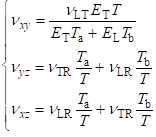(3)

where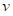LT,TR, andLR are the Poisson’s ratios in the LT, TR, and LR planes of the veneer, respectively

Beam specimens were cut from the plywood panel, as shown in Fig. 1a. A speci-men with a length direction coincident with the longitudinal direction of the surface veneer is defined as an L-type specimen, whereas one with a length direction coincident with the tangential direction of the surface veneer is defined as a T-type specimen. For a beam specimen cut from the plywood panel, the length, width, and thickness directions are defined as the XY, and Z directions, respectively. Therefore, the X-axis of the L- and T-type specimens coincides with the xand y axes of the plywood panel, respectively.

FINITE ELEMENT ANALYSIS (FEA)

Three-dimensional (3D) FEA was performed independently of the flexural vibration test using the FEA program ANSYS 12.0 (2009). Figure 2 shows the FE mesh of the specimen. In the analyses, the following four different constructions were simulated: 3-ply, 5-ply, 7-ply, and solid models. The solid model was assumed to be homogeneous, and the elastic constants were obtained by substituting the elastic constants of the veneers into Eqs. 1 through 3. The model had a length, L, of 300 mm. The depth, H, was varied from 10 to 60 mm at 10 mm increments. The width T, which corresponds to the total thickness of the plywood panel, was given values of 6, 9, and 12 mm, and the thickness of each veneer was determined as shown in Table 1.

Table 1. Construction of Plywood Models Used in the Finite Element Analyses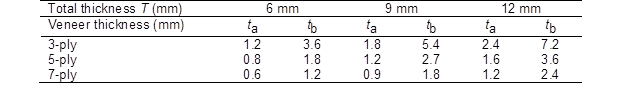ta and tb are the thicknesses of the surface veneer and the veneer adjacent to the surface veneer, respectively.

The model consisted of eight-noded brick elements. The ratio Tb/Ta was always constant and equal to 1.5 in these constructions.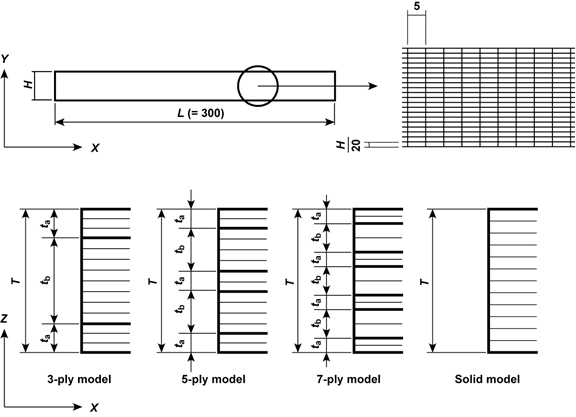Fig. 2. Finite element model for the plywood beams shown in the XY and XZ planes

Table 2 shows the derived elastic constants of Lauan (Shorea sp.) for the veneer and those derived for the solid model used in the calculations. It was difficult to measure the elastic constants of the veneer that the actual plywood panel was composed of. Therefore, the elastic constants of the veneer in Table 2 were taken from the data published in the Wood Industry Handbook (Forestry and Forest Products Research Institute 2004).

Table 2. Elastic Properties Used for the Finite Element Analysis Calculations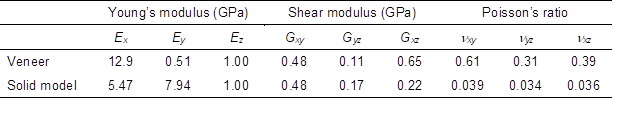xy, and z of the veneer represent the longitudinal, tangential, and radial directions, respectively. Properties of the solid model are derived from Eqs. (1)-(3) are based on classical lamination theory.

As described above, the elastic constants for the solid model were calculated by substituting the elastic constants of Lauan into Eqs. (1)-(3). The density of the model was 500 kg/m3. In the analyses, the following two models were simulated in terms of wood grain: (a) the length direction of the surface veneer equals longitudinal direction, and (b) length direction of the surface veneer equals tangential direction. These models were defined as the L- and T-type models, respectively, and their definitions are similar to those described above.

Modal analyses were conducted, and the resonance frequencies from the 1st to 4th flexural vibration modes were extracted; the Young’s modulus and the in-plane shear modulus were determined from the solution of Timoshenko’s differential equation (1921) as follows: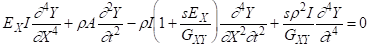(4)

where EX is the Young’s modulus in the length (X) direction of the model, GXY is the in-plane shear modulus in the XY plane, I is the secondary moment of inertia, A is the cross-sectional area,  is the density, and s is Timoshenko’s shear factor. The solution of Eq. (4) under the free-free flexural condition derived by Goens is (1931),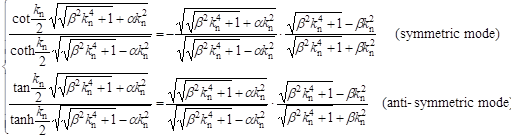(5)

where n is the mode number, and  and  are derived using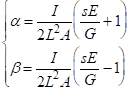(6)

When the resonance frequency for the nth flexural vibration mode is defined as fnkn is derived using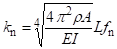(7)

Both the EX and GXY/s terms can be derived from the solution, the GXY/s values corresponding to each vibration mode were calculated by altering the value of EX, and the coefficient of variation (COV) of the GXY/s values was determined. The EX value that generates the minimum COV of the GXY/s values, and the mean value of GXY/s can be regarded as being the most feasible. Approximation solutions were obtained using Mathematica 6 (2007). By referring to several previous investigations (Hearmon 1958; Divós et al. 1998, 2005), the value of s was provisionally determined to be 1.2 in this analysis. This method was similar to that adopted in previous works (Yoshihara 2011; 2012).

According to lamination theory, the EX values should be 5.47 and 7.94 GPa for the L- and T-type models, respectively, which correspond to the Ex and Ey values, respectively. These values are shown in Table 2 independently of the plywood constructions. The value of GXY should be 0.48 GPa for both models, which corresponds to Gxy in Table 2.

EXPERIMENTAL

Specimens

Lauan wood (Shorea sp.) with 3-, 5-, and 7-ply constructions, which were 6, 9, and 12 mm thick, respectively, were investigated. The samples were fabricated by Ueno Mokuzai Kogyo Co. (Himeji, Japan) and had initial lengths and widths of 1820 and 910 mm, respectively. In the FEAs, nine different constructions corresponding to different model thicknesses and ply numbers were investigated as shown in Table 1. In the actual vibration tests of ordinary commercial plywood, however, it was difficult to prepare the materials with such varied constructions as those adopted for the FE models. Table 3 shows the thicknesses of the veneers and the densities of each plywood panel. Although the constructions of the actual plywood samples were different from those adopted for the FE models, we can evaluate the influence of plywood construction and specimen configuration. The plywood samples were stored in a room at a constant temperature of 20C and relative humidity of 65% to reach an equilibrium moisture content (EMC) of approximately 12%.

Table 3. Thickness of the Veneer of the Plywood Used for the Actual Vibration Tests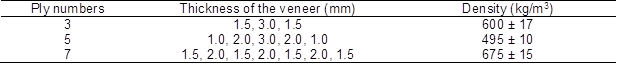Results of the densities are averages ± SD.

The L-type specimens had initial length and depth dimensions of 300 (X = x) 60 (Y = y) mm2, whereas the T-type specimens had initial length and depth dimensions of 300 (X = y) 60 (Y = x) mm2. Ten specimens were prepared for each type, and the densities shown in Table 3 were measured using these twenty specimens with the initial dimensions. After conducting the flexural vibration tests described below, the depth of the specimen was reduced, and the succeeding series of vibration tests was conducted using the specimens with reduced depth. The depth (H) of the specimens was reduced from 60 to 10 mm in intervals of 10 mm.

Flexural Vibration Test

Figure 3 shows diagram of the flexural vibration test. The specimen was suspended by threads at the nodal positions of the free-free resonance vibration mode fn and excited in the depth direction (Y) with a hammer. It was difficult to measure resonance frequencies above the 5th mode in the vibration test because the amplitude of the vibration was small in the high-frequency modes. In addition, it was often difficult to distinguish the vibrations caused by the flexural mode from those caused by other modes in the high-frequency range. Thus, the resonance frequencies of the 1st to 4th mode were measured in this investigation. The resonance frequencies were analyzed using a fast Fourier transform (FFT) analysis program. Similar to the FEA method, the EX and GXY values were calculated using Mathematica 6. This method was similar to that adopted in previous works (Yoshihara 2011; 2012). As described above, the EX values obtained using the L- and T-type specimens correspond to Ex and Ey of the plywood panel, respectively, whereas GXY corresponds to Gxy of the plywood panel for both types.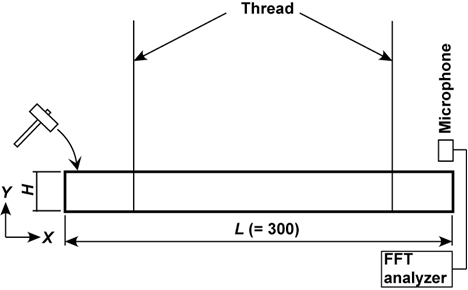Fig. 3. Diagram of the flexural vibration test of a specimen. Unit = mm. H varies from 10 to 60 mm with an interval of 10 mm. Specimen is supported by threads located at the most outer positions of vibration modes. The definitions of X and Y are shown in Fig. 1.

RESULTS AND DISCUSSION

Finite Element Analyses

Figure 4 shows the dependence of the Young’s moduli Ex and Ey obtained by FEA on the depth/length ratio (H/L). In a previous work on flexural vibration tests of MDF, the FEAs indicated that the Young’s modulus was not dependent on the H/L (Yoshihara 2011). In contrast, the Young’s modulus of plywood tends to increase as the H/L ratio decreases, except for a few cases. Nevertheless, the variation of the Young’s modulus is not more significant than that of the shear modulus, the details of which are described below.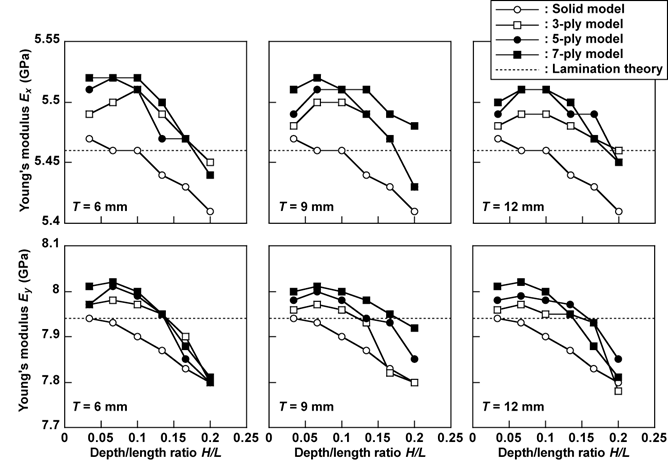Fig. 4. Dependence of the Young’s moduli (Ex and Ey) on the depth/length ratio (H/L) corresponding to the ply numbers and total thickness of the plywood model (T) obtained by FEA

Figure 5 shows the dependence of the in-plane shear modulus Gxy on the H/L ratio, as determined by FEA. In comparison to the Ex and Ey values, the Gxy value is strongly dependent on the H/L ratio. The Gxy value tends to decrease as the H/L ratio decreases, except for a few results. This decreasing trend is opposite to that observed in the analyses of MDF, where the in-plane shear modulus increased as the H/L decreased (Yoshihara 2011). This trend is more pronounced as the ply number decreases and the thickness of plywood T increases, which corresponds to the width of the FE model. Since the FEA results for the MDF and solid models, which can be regarded as being homogeneous, do not show the decreasing trend, the inhomogeneity due to lamination construction has a significant influence on the decreasing of Gxy as the H/L decreases.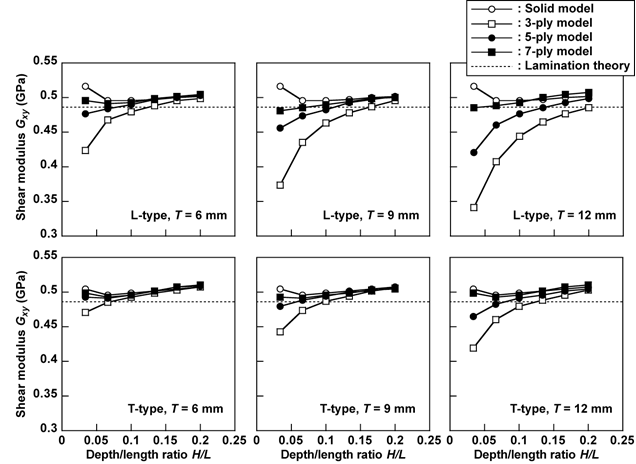Fig. 5. Dependence of the in-plane shear modulus (Gxy) on the depth/length ratio (H/L) corresponding to the ply numbers and total thickness of the plywood model obtained by FEA

Flexural Vibration Tests

Figure 6 shows the Ex and Ey values obtained experimentally from flexural vibration tests. Statistical analysis of the difference between the Young’s moduli of specimens with different H/L ratios showed that the difference was not significant over the entire range of H/L because all probability values (P-value) obtained by comparison with the corresponding values of Young’s modulus were larger than the significance level of 0.05.

Figure 7 shows a comparison of the Gxy values determined from the flexural vibration tests. In contrast to the results obtained from the flexural vibration tests of MDF (Yoshihara 2011), the value of Gxy decreases with decreasing H/L. A statistical analysis among the Gxy values calculated for various depth/length ratios determined that the Gxy values for the specimens with an H/L ratio of 0.033 (corresponding to a depth of 10 mm) are smaller than those with larger values of H/L, at a significance level of 0.01. The only exception is the T-type specimen of 7-ply plywood, in which the influence of H/L on the Gxy value is not significant. When the H/L ratio is 0.067 (corresponding to a depth of 20 mm), the Gxy values for the L- and T-types of 5-ply plywood are smaller than those obtained for larger values of H/L with significance levels of 0.01 and 0.05, respectively.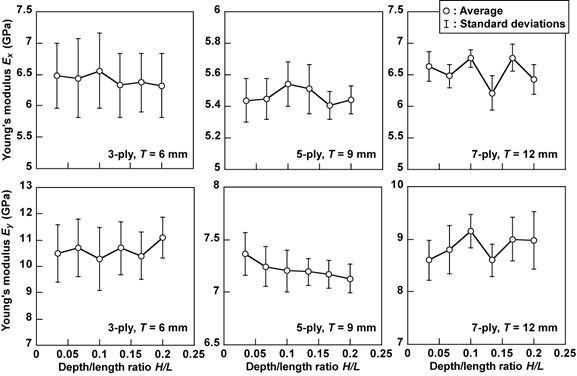Fig. 6. Young’s moduli (Ex and Ey) obtained by the flexural vibration tests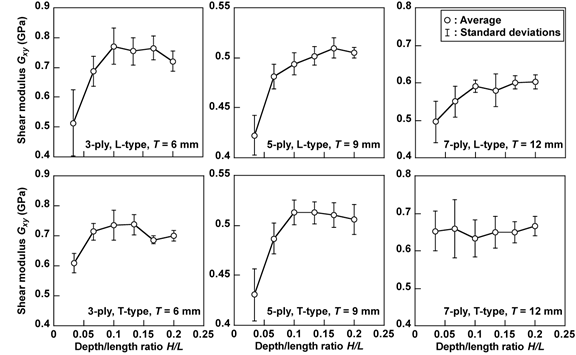Fig. 7. Shear modulus (Gxy) obtained by the flexural vibration tests

For specimens with H/L larger than 0.1 (corresponding to depths larger than 30 mm), there are no significant differences among the Gxy values. These statistical results suggest that the depth H should be larger than 30 mm for specimens with lengths, L, of 300 mm (which corresponds to 10 times the depth) to obtain the reliable in-plane shear modulus while reducing the influence of H/L.

As described above, the Young’s modulus obtained from the FEA tends to increase slightly as the H/L ratio decreases. Therefore, the increasing trend in the experimental results is obscured due to the variation of the Young’s modulus values corresponding to the H/L ratio. In contrast, the in-plane shear modulus obtained from the FEA tends to decrease as the H/Lratio decreases, and the decreasing trend is more pronounced as the ply number decreases and the total thickness of plywood T increases. For the T-type model of 7-ply plywood, however, the decreasing trend is not so significant. These trends are commonly found in the experimental results, although the constructions of the models are different from those of the plywood panels used for the actual vibration tests. From the results of FEA and experiment, it is obvious that the depth to length ratio should be carefully determined for determining the in-plane shear modulus of plywood while considering its construction.

In previous studies on MDF and solid wood, Timosheko’s shear factor, s, was analyzed numerically to improve the accuracy in the measurement of the shear modulus, and it was determined that the configuration and orthotropy of the specimen have a significant influence on the s value (Yoshihara 2011; 2012). For plywood, however, the lamination construction has a large influence in addition to these factors. Therefore, it will be more complex to determine the s value while considering the influence of the lamination construction in addition to the specimen configuration, although the range of H/L that is relevant for the determination of the in-plane shear modulus may be expanded. To obtain the in-plane shear modulus practically, it is preferable to restrict the range of H/L while substituting 1.2 for s.

Based on this experiment, the Young’s modulus/in-plane shear modulus ratio ranges from approximately 8 to 15. For solid wood, which also has a Young’s modulus/shear modulus ratio in this range, these moduli can be measured accurately when using specimens with a length that is more than 30 times greater than their depth (Kubojima et al. 1997). For the plywood examined here, however, the in-plane shear modulus decreases as the depth/length ratio decreases because of the lamination construction of the material. A statistical analysis reveals that the difference between the Gxy values was not significant when the depth exceeds 30 mm for specimens with lengths that were 10 times greater than their depths. Therefore, the specimen length should be smaller than 10 times the depth to accurately measure the in-plane shear modulus of plywood based on Timoshenko’s vibration theory. Nevertheless, the mechanical properties of plywood vary according to its construction including the ply number, thicknesses of the veneers, and species used for the veneers. The specimen configuration appropriate for measuring the elastic properties may also vary according to the plywood construction. Therefore, further research is required to measure the elastic properties of various kinds of plywood fabricated under various conditions via vibration tests.

CONCLUSIONS

Lauan plywood samples with 3-, 5-, and 7-ply constructions were tested to determine the Young’s modulus and in-plane shear modulus. The flexural vibration test method was experimentally and numerically employed. The conclusions are summarized as follows:

1. The experimental and FEA results indicate that valid Young’s modulus values can be obtained by the flexural vibration method.
2. The FEA results suggest that the in-plane shear modulus tends to decreases as the depth/length ratio decreases. This decreasing trend is opposite to that observed in the analyses of MDF in an earlier study (Yoshihara 2011). This trend is more pronounced as the ply number of the plywood decreases and its thickness increases. The experimental results also show the same trend obtained from the FEA.
3. The experimental results indicate that the influence of the specimen configuration on the measurement of the in-plane shear modulus can be effectively reduced when the length of the specimen is less than 10 times the depth.

REFERENCES CITED

Adams, D. F., Carlsson, L. A. and Pipes, R. B. (2003). Experimental Characterization of Advanced Composite Materials, 3rd Ed., CRC Press, Boca Raton.

ANSYS (2009). Release 12.0. Canonsburg, PA: Ansys Inc.

Brancheriau, L. (2006). “Influence of cross section dimensions on Timoshenko’s shear factor. Application to wooden beams in free-free flexural vibration,” Ann. Forest Sci. 63, 319-322.

Brancheriau, L., and Baillères, H. (2002). “Natural vibration analysis of clear wooden beams: A theoretical review,” Wood Sci. Technol. 36, 347-365.

Brancheriau, L., and Baillères, H. (2003). “Use of the partial least squares method with acoustic vibration spectra as a new grading technique for structural timber,” Holzforschung 57, 644-652.

Chui, Y. H., and Smith, I. (1990). “Influence of rotatory inertia, shear deformation and support condition on natural frequencies of wooden beams,” Wood Sci. Technol. 24, 233-245.

Divós, F., Tanaka, T., Nagao, H., and Kato, H. (1998). “Determination of shear modulus on construction size timber,” Wood Sci. Technol. 32, 393-402.

Divos, F., Denes, L., and Iñigues, G. (2005). “Effect of cross-sectional change of a board specimen on stress wave velocity determination,” Holzforschung 59, 230-231.

Forestry and Forest Products Research Institute, Japan ed. (2004). “Wood Industry Handbook,” Maruzen, Tokyo.

Goens, E. (1931). “Über die Bestimmung des Elastizitätsmodulus von Stäben mit Hilfe von Biegungsschwingungen,“ Ann. Phys. Ser. 7(11), 649-678.

Haines, D. W., Leban, L. M., and Herbé, C. (1996). “Determination of Young’s modulus for spruce, fir and isotropic materials by the resonance flexure method with comparisons to static flexure and other dynamic methods,” Wood Sci. Technol. 30, 253-163.

Hearmon, R. F. S. (1946). “The fundamental frequency of vibration of rectangular wood and plywood plate,” Proc. Phys. Soc. 58, 78-92.

Hearmon, R. F. S. (1948). “Elasticity of wood and plywood,” HM Stationary Office, London.

Hearmon, R. F. S. (1958). “The influence of shear and rotatory inertia on the free flexural vibration of wooden beams,” Brit. J. Appl. Phys. 9, 381-388.

Hearmon, R. F. S. (1966). “Vibration testing of wood,” Forest Prod. J. 16(8), 29-40.

Kubojima, Y., Yoshihara, H., Ohta, M., and Okano, T. (1996). “Examination of the method of measuring the shear modulus of wood based on the Timoshenko theory of bending,” Mokuzai Gakkaishi, 42, 1170-1176.

Kubojima, Y., Yoshihara, H., Ohta, M., and Okano, T. (1997). “Accuracy of the shear modulus of wood obtained by Timoshenko theory of bending,” Mokuzai Gakkaishi, 43, 439-443.

Mathematica 6. Champaign, IL: Wolfram Research; 2007.

Murata, K., and Kanazawa, T. (2007). “Determination of Young’s modulus and shear modulus by means of deflection curves for wood beams obtained in static bending tests,” Holzforschung 61, 589-594.

Nakao, T. (1984). “Measurement of the anisotropic-shear modulus by the torsional vibration method for free-free wooden beams,” Mokuzai Gakkaishi 30, 877-885.

Nakao, T. and Okano, T. (1987). “Evaluation of modulus of rigidity by dynamic plate shear testing,” Wood Fiber Sci. 19, 332-338.

Sobue, N. (1986). “Instantaneous measurement of elastic constants by analysis of the tap tone of wood: Application to flexural vibration of beams,” Mokuzai Gakkaishi 32, 274-279.

Sohi, A. M. A., Khademi-Eslam, H., Hemmasi, A. H., Roohnia, M., and Talaipour, M. (2011). “Nondestructive detection of the effect of drilling of acoustic performance of wood,” BioResources 6, 2632-2646.

Takami, I. (1964). “Poisson’s ratio of plywood,” Bull. Gov. Forest Exp. Sta. 49, 133-174.

Timoshenko, S. P. (1921). “On the correction for shear of the differential equation for transverse vibrations of prismatic bars,” Phil. Mag. 41, 744-746.

Tonosaki, M., Saito, S. and Miyamoto, K. (2010). “Evaluation of internal checks in high temperature dried sugi boxed heart square sawn timber by dynamic shear modulus,” Mokuzai Gakkaishi 56, 79-83.

Yoshihara, H. (2009a). “Edgewise shear modulus of plywood measured by square-plate twist and beam flexure methods,” Construct. Build. Mater. 23, 3537-3345.

Yoshihara, H. (2009b). “Poisson’s ratio of plywood measured by tension test,” Holzforschung 63, 603-608.

Yoshihara, H. (2010). “Measurement of the in-plane shear modulus of plywood by tension test of  off-axis specimen,” Trans. Jpn. Soc. Mech. Eng. A 77, 670-678.

Yoshihara, H. (2011). “Measurement of the Young’s modulus and shear modulus of in-plane quasi-isotropic medium-density fiberboard by flexural vibration,” BioResources 6, 4871-4885.

Yoshihara, H. (2012). “Off-axis Young’s modulus and off-axis shear modulus of wood measured by flexural vibration tests,” Holzforschung 65, in press.

Article submitted: November 16, 2011; Peer review completed: December 16, 2011; Revised version received: December 21, 2011; Accepted: January 26, 2012; Published: January 28, 2012.